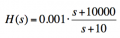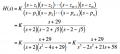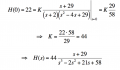# Transfer function of OpAmp

#### sailorjoe

Joined Jun 4, 2013
363
Yes, Emil, if s = infinity, you plug that in and solve for the gain or the transfer function.
Basic algebra.

Predicting the gain just by looking at the circuit is a skill that comes with an understanding of circuit theory and some practice. This particular problem is a good example of how it works. But first you have to have a firm grasp of the concept of impedance as a function of frequency. If you don't fully understand that, then you can't understand what all the parts in the circuit are doing. In that case, just stick with the the math and work it out.

Thread Starter

#### Emil Skovgaard

Joined Jun 6, 2015
37
I understand. Their were similar assignments in some of the supplementary material, so i got a better understanding after solving them, so thanks!

Now.. onto something which is rather new for me - sketching, or drawing.
Still with addition to the transfer function and this OpAmp circuit, i'm asked to (sorry for the maybe bad translation) sketch the frequency response, both amplitude- and the phase response i curves.
Hoping that some can guide me here?

Thread Starter

#### Emil Skovgaard

Joined Jun 6, 2015
37
Nevermind, it got the hang of sketching amplitude- and phase responsesIn addition to the transfer function, the circuit is to be changed so a DC gain on 20dB results - how do i accomplish that? I can't seem do find anything upon the subject?

#### Jony130

Joined Feb 17, 2009
5,230
But you already should know the answer, what is the gain for s=0?

Thread Starter

#### Emil Skovgaard

Joined Jun 6, 2015
37
The gain for s=0 is -R2/R1. So if i want i gain of 20dB, do i insert the 20, or how do i come around it?

#### Jony130

Joined Feb 17, 2009
5,230
dB is a logarithmic representation of a ratio. So 20dB is not equal to 20V/V

Thread Starter

#### Emil Skovgaard

Joined Jun 6, 2015
37
Yes, i'm away of that.

I shall show that gain of 2dB simply can be obtained, and make a new transfer function. While it's still in addition to a transfer function, this one is different (not that it matters muchInstead of H(s) being =, would i just input the 20dB, because that is what is wanted now, so it's 20dB = (the equation)?

EDIT:
Isn't it just inserting 20dB on s place (omega), and something about having a K in front of the equation?

#### Jony130

Joined Feb 17, 2009
5,230
But for DC the "s" is equal to zero and ω is also zero.

#### MrAl

Joined Jun 17, 2014
8,251
The gain for s=0 is -R2/R1. So if i want i gain of 20dB, do i insert the 20, or how do i come around it?

If you know that the gain is R2/R1 and you know it should be 20db, then what would you do from there?
Make an equation that describes this, then solve.

20=20*log(R2/R1)

where 'log' is the log base 10 .

Not to difficult right? Now solve that for either R1 or R2 or both, or just the ratio R2/R1.

Thread Starter

#### Emil Skovgaard

Joined Jun 6, 2015
37
I see, but that gain, or the used equation is when s = 0, so i don't know if i can use that as a starting point?

I had a similar assignment, where i had a transfer, and i wanted a DC gain of 22Isn't this the same way i should solve this problem? Insert the K in front of the equation, in this case 20, and solve it for K, and put in front? Or is this a whole other topic? It just seems similar

#### Jony130

Joined Feb 17, 2009
5,230
LOL, but you already solve H(s) for s-->0 and the result is -R2/R1. Why are you making the simple things so complicated?

Thread Starter

#### Emil Skovgaard

Joined Jun 6, 2015
37
I know i might fix a lot og things together, i just think theres so many equations and theory leading to similar results (like the one i posted)
But thanks for the help, it is much appreciated#### MrAl

Joined Jun 17, 2014
8,251
Hi,

Well, assuming you know the DC gain is R2/R1 already and you want to change R2 or R1 or whatever, then you would do G=20*log(R2/R1), but if you prefer to find a multiplicative gain then you could do:

G=20*log(K*R2/R1)

and then solve for K instead of R1 or R2.

This would be the case when you want to introduce another completely different gain stage (K) into the circuit, not adjust either R1 or R2.

This is also assuming that you want to adjust the DC gain. If you want to adjust the gain at some frequency F, then you have to keep s=j*w in the equation and solve for that gain instead, then adjust or whatever.
This would be the case when you have for example a band pass filter, where you want to adjust the gain in the pass band (very common). You would first solve for the gain in the pass band and then adjust that instead. Solving for the gain in the pass band in that case would involve finding the frequency Fo where the gain is the highest, then using that frequency as the frequency to be used for adjusting the gain. Sometimes that is easy to calculate sometimes a bit harder to do depending on the complexity of the circuit which usually depends on how many inductors and capacitors are in the circuit.
For a band stop filter you would first solve for frequency that causes the lowest gain in the stop band, then use that as the frequency for adjusting the gain.
So it partly depends on the filter type, and it may get more complicated depending on how complex the response is too. For the simpler filters though it's not that hard to do:

For H(s)=H(jw)=Hjw:
0=d(Hjw)/dw

solve that for all critical w, then figure out which one you want to use as w0 and that would then be used as the frequency at which to adjust the gain.

Last edited:
Similar threads